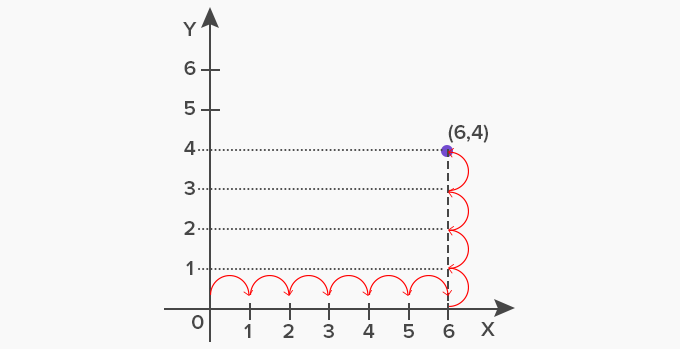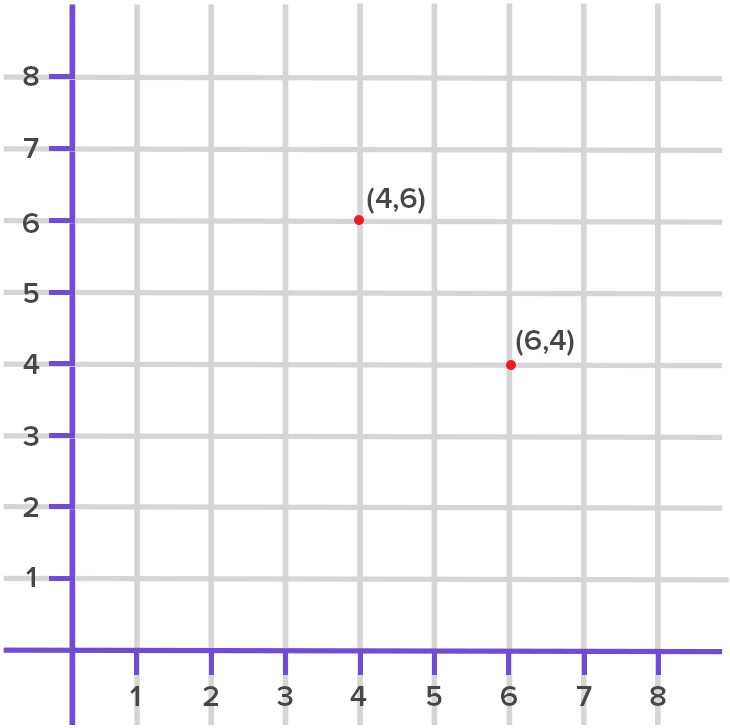# Ordered Pair - Definition with Examples

The Complete K-5 Math Learning Program Built for Your Child

• 30 Million Kids

Loved by kids and parent worldwide

• 50,000 Schools

Trusted by teachers across schools

• Comprehensive Curriculum

Aligned to Common Core

## Ordered Pair

An ordered pair is a composition of the x coordinate (abscissa) and the y coordinate (ordinate), having two values written in a fixed order within parentheses.

It helps to locate a point on the Cartesian plane for better visual comprehension.

The numeric values in an ordered pair can be integers or fractions.

 Ordered Pair = (x,y)   Where, x = abscissa, the distance measure of a point from the primary axis “x”   And, y = ordinate, the distance measure of a point from the secondary axis “y”

In the Cartesian plane, we define a two-dimensional space with two perpendicular reference lines, namely x-axis and y-axis. The point where the two lines meet at “0” is the origin.

To comprehend it better, let’s take an example. Plot the point “P” with coordinates 6, 4.

As per the definition of ordered pair, the point P will be written as:

• P = (6, 4)
• The first number in the ordered pair shows the distance from “x" axis which is 6
• The second number in the ordered pair shows the distance from “y" axis which is 4

To mark the point on the Cartesian plane, start from the origin. Take 6 steps towards the “x” axis (towards right) starting from the origin. From here, take 4 steps towards the “y” axis (upwards).As the name “ordered pair” suggests, the order in which values are written in a pair is very important. The ordered pair (6, 4) is different from the pair (4, 6). Both represent two different points as shown below.Application

• The concept of ordered pair is highly useful in data comprehension as well for word problems and statistics.
• The coordinate geometry uses ordered pairs to represent geometric figures and objects in an open space for visual comprehension. Geometric shapes like circle, triangle, square, rectangle and polygons use the ordered pairs to represent the center, vertices and the length of the sides with coordinates.

 Fun facts The mathematician Rene Descartes and Pierre de Fermat invented analytic geometry in 16th century and Cartesian plane was designed Ordered pair in modern math is widely used in the field of computing and programming languages

Won Numerous Awards & Honors